# C Program to Print the Table of a Number

In this tutorial, you will learn and get code for printing the table in the following ways:

## Print a Table of a Specific Number in C

To print the table of any number in C programming, you have to ask the user to enter any number. Then multiply the number by 1 to 10 and display the multiplication result when multiplying the number by 1, 2, 3,... 9, 10 as shown in the program below.

The question is: write a program in C to receive any number from the user (at run-time) and print its table. Here is the answer to this question:

```#include<stdio.h>
#include<conio.h>
int main()
{
int num, i, tab;
printf("Enter the number: ");
scanf("%d", &num);
printf("\nTable of %d is:\n", num);
for(i=1; i<=10; i++)
{
tab = num*i;
printf("%d * %2d = %2d\n", num, i, tab);
}
getch();
return 0;
}```

Because the program was written in the Code::Blocks IDE, you will see the following output after a successful build and run: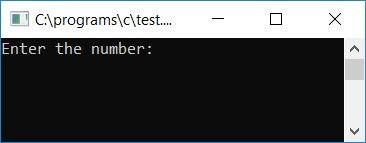Supply any number, say 5, and press the ENTER key to see the table of 5 as given in the second snapshot of the sample run: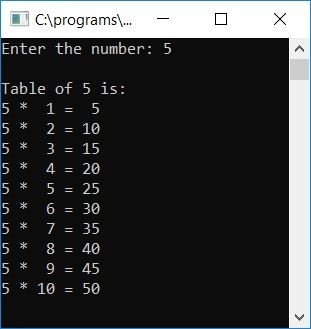#### Program Explained

• Receive any number as input, say 5, to print the table of 5.
• Make a for loop that goes from 1 to 10.
• Inside the for loop, multiply the number with the value of i and initialize it to another variable, say tab.
• Then print the value of tab as output each and every time after multiplication and initialization inside the for loop.
• Here, i holds 1 for the first time, and then tab holds num*i or 5*1 or 5.
• The second time, i will hold 2 and tab will hold num*i, 5*2, or 10.
• In a similar way, "tab" will hold 5, 10, 15,... 45, 50.

## Print Tables 1 through 10 in C

This program will print Table 1 through Table 10.

```#include<stdio.h>
#include<conio.h>
int main()
{
int num=1, res, i, j;
printf("\t\tTable from 1 to 10\n\n");
for(i=0; i<10; i++)
{
printf("Table of %d = ", num);
for(j=1; j<=10; j++)
{
res = num*j;
printf("%d  ", res);
}
printf("\n");
num++;
}
getch();
return 0;
}```

Here is its sample run: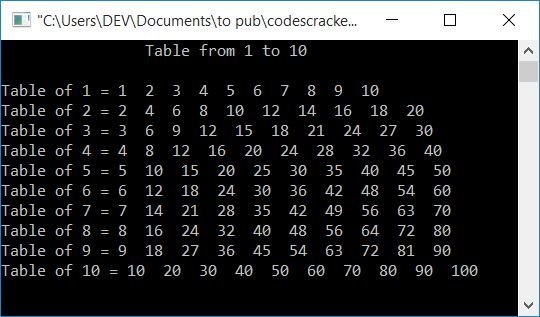## C Print the Table in the Given Range

Let's create another program that will also print the table of numbers. But this time, the program will ask for the range (starting and ending numbers). The table of all the numbers that come in between these two given numbers (including both) will be printed as output.

```#include<stdio.h>
#include<conio.h>
int main()
{
int n1, n2, i, j;
printf("Enter the value of n1 (starting point): ");
scanf("%d", &n1);
printf("Enter the value of n2 (ending point): ");
scanf("%d", &n2);
for(i=n1; i<=n2; i++)
{
printf("Table of %d:\n", i);
for(j=1; j<=10; j++)
{
printf("%d x %d = %d\n", i, j, i*j);
}
printf("\n");
}
getch();
return 0;
}```

Here is the first snapshot of the sample run: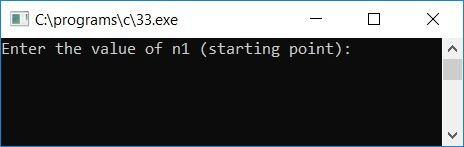Now supply any two numbers, say 2 and 3, as the starting and ending points, and press ENTER to see the output. Tables 2 and 3 will be the result: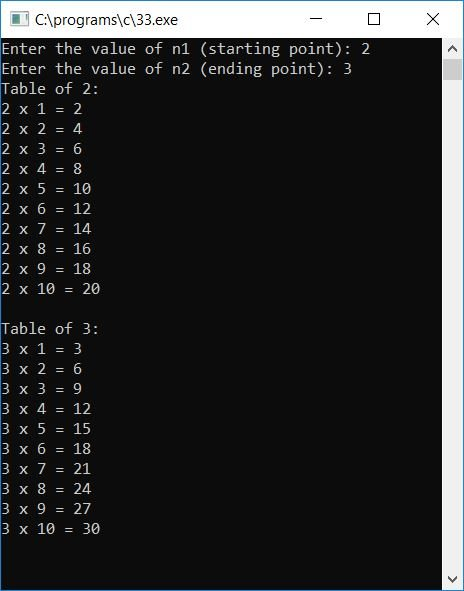#### The same program in different languages

C Quiz

« Previous Program Next Program »